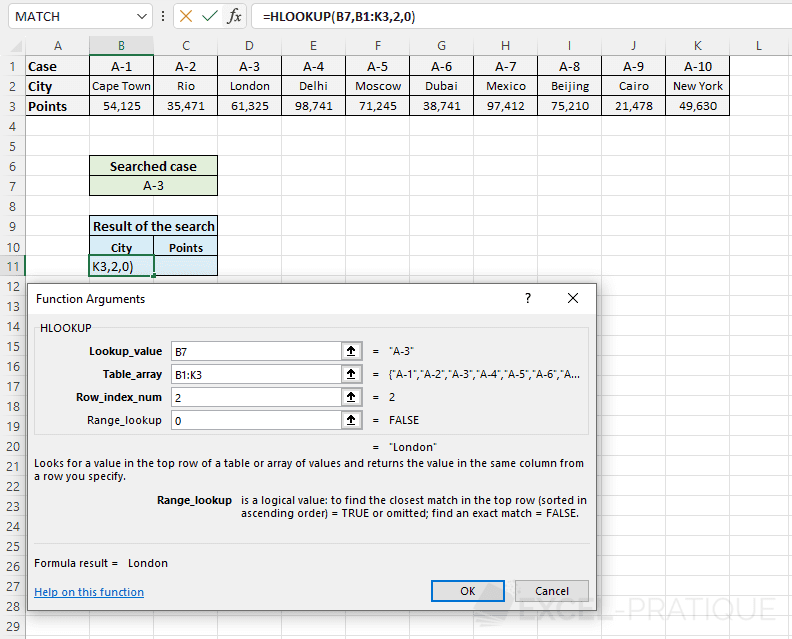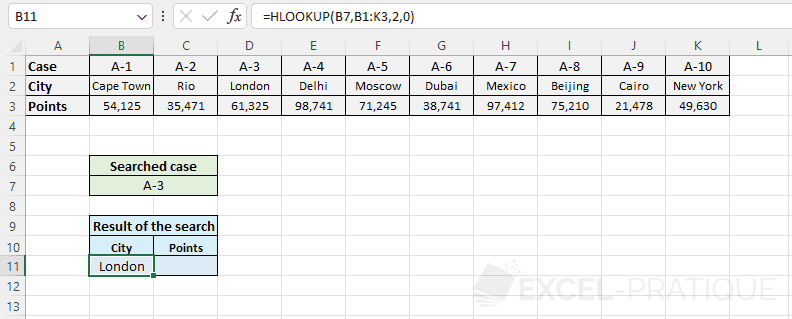# Excel function HLOOKUP

The HLOOKUP function finds a value in the first row of a table and then returns the contents of a cell in the same column.

Let's try an example:

We will use HLOOKUP to display a person's age based on his or her fist name.

In this example, we want Steve's age.

• In the "Lookup_value" field, we'll enter C5, which is the cell that contains Steve's name.
• In the "Table_array" field, we will enter a data range that contains the table.
• In the "Row_index_num" field, we have to enter the number of the row that contains the data to be displayed (in this case, the ages). The row number refers to the row's number within the table defined in "Table_array".
• The "Range_lookup" field is optional. It allows you to search based on the exact value shown in "Lookup_value" (by entering: FALSE), or based on the closest value to "Lookup_value" (by entering: TRUE or leaving the field empty).And now we have retrieved Steve's age.

Now all we have to do is edit C5 to retrieve a different person's age.